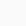# Wiley’s Mathematics for JEE Trigonometry, Vector Algebra, Probability

PDF Free Download | Wiley s Mathematics for IIT JEE Main and Advanced Trigonometry Vector Algebra Probability Vol 2

## Contents of Mathematics for JEE eBook

• Trigonometric Ratios and Transformations
• Angles and Their Measures
• Trigonometric Ratios
• Periodicity and Variance
• Trigonometric Ratios of Compound Angles
• Trigonometric Ratios of Multiple and Submultiple Angles
• Sum and Product Transformations
• Worked-Out Problems
• Summary
• Exercises
• Inverse Trigonometric Functions
• Domains and Ranges of Inverse Trigonometric Functions
• Properties of Inverse Trigonometric Functions
• Worked-Out Problems
• Summary
• Exercises
• Trigonometric Equations
• The Least Positive Angles with a Given Trigonometric Ratio
• General Solution of Equations of the Form sinx = a
• Solutions of Simple Trigonometric Equations
• Worked-Out Problems
• Summary
• Exercises
• Properties of Triangles
• Relations Between the Sides and the Trigonometric Ratios of Angles of a Triangle
• Solution of Triangles
• Properties of a Triangle
• Worked-Out Problems
• Summary
• Exercises
• Addition and Scalar Multiplication of Vectors
• Definition and Classification of Vectors
• Addition of Vectors
• Multiplication of a Vector by a Scalar
• The Division Formula
• Components of a Vector
• Vector Equation of a Line and a Plane
• Worked-Out Problems
• Summary
• Exercises
• Multiplication of Vectors
• Scalar or Dot Product
• Vector Equations of a Plane and a Sphere
• Vector or Cross Product
• Vector Areas
• Scalar Triple Product
• Vector Triple Product
• Worked-Out Problems
• Summary
• Exercises
• Probability
• Random Experiments and Events
• Classical Definition of Probability
• Axiomatic Approach to Probability
• Independent and Dependent Events
• Random Variables and Probability Distributions
• Theoretical Discrete Distribution
• Worked-Out Problems
• Summary
• Exercises
• Inequalities
• Introduction
• Worked-Out Problems
• Exercises
Share book:

## Related Free BooksAuthor

Language

Pages

Size

Format

Category

# Wiley’s Mathematics for JEE Trigonometry, Vector Algebra, Probability

## Related BooksWhat's the problem with this file?# Attention !

## Help us remove any copyrighted material that may be posted on our site.

We ask you to inform us of any infringing material that may be unintentionally published on our site to remove it.

Reach us at: [email protected]

# Attention !

## Help us remove any copyrighted material that may be posted on our site.

We ask you to inform us of any infringing material that may be unintentionally published on our site to remove it.

Reach us at: [email protected]